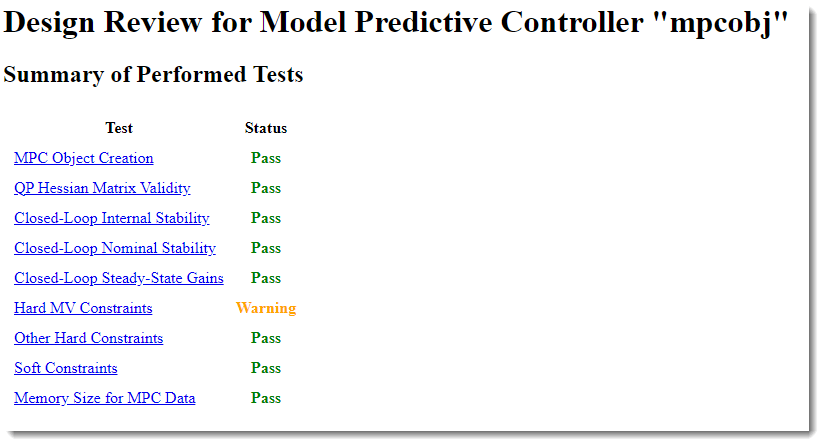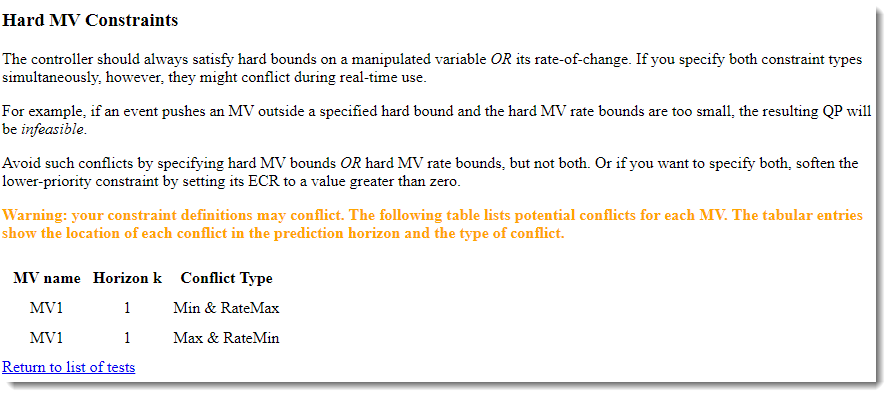# review

Examine MPC controller for design errors and stability problems at run time

## Syntax

``review(mpcobj)``
``results = review(mpcobj)``

## Description

example

````review(mpcobj)` checks for potential design issues in the model predictive controller, `mpcobj`, and generates a testing report. The testing report provides information about each test, highlights test warnings and failures, and suggests possible solutions. For more information on the tests performed by the `review` function, see Algorithms.```

example

````results = review(mpcobj)` returns the test results and suppresses the testing report. ```

## Examples

collapse all

Define a plant model, and create an MPC controller.

```plant = tf(1, [10 1]); Ts = 2; MPCobj = mpc(plant,Ts);```
```-->The "PredictionHorizon" property of "mpc" object is empty. Trying PredictionHorizon = 10. -->The "ControlHorizon" property of the "mpc" object is empty. Assuming 2. -->The "Weights.ManipulatedVariables" property of "mpc" object is empty. Assuming default 0.00000. -->The "Weights.ManipulatedVariablesRate" property of "mpc" object is empty. Assuming default 0.10000. -->The "Weights.OutputVariables" property of "mpc" object is empty. Assuming default 1.00000. ```

Set hard upper and lower bounds on the manipulated variable and its rate of change.

```MV = MPCobj.MV; MV.Min = -2; MV.Max = 2; MV.RateMin = -4; MV.RateMax = 4; MPCobj.MV = MV;```

Review the controller design. The review function generates and opens a report in the Web Browser window.

`review(MPCobj)`
```-->Converting the "Model.Plant" property of "mpc" object to state-space. -->Converting model to discrete time. -->Assuming output disturbance added to measured output channel #1 is integrated white noise. -->The "Model.Noise" property of the "mpc" object is empty. Assuming white noise on each measured output channel. ````review` flags a potential constraint conflict that could result if this controller was used to control a real process. To view details about the warning, click Hard MV Constraints.Define a plant model, and create an MPC controller.

```plant = rss(3,1,1); plant.D = 0; Ts = 0.1; MPCobj = mpc(plant,Ts);```
```-->The "PredictionHorizon" property of "mpc" object is empty. Trying PredictionHorizon = 10. -->The "ControlHorizon" property of the "mpc" object is empty. Assuming 2. -->The "Weights.ManipulatedVariables" property of "mpc" object is empty. Assuming default 0.00000. -->The "Weights.ManipulatedVariablesRate" property of "mpc" object is empty. Assuming default 0.10000. -->The "Weights.OutputVariables" property of "mpc" object is empty. Assuming default 1.00000. ```

Specify constraints for the controller.

```MV = MPCobj.MV; MV.Min = -2; MV.Max = 2; MV.RateMin = -4; MV.RateMax = 4; MPCobj.MV = MV;```

Review the controller design, and suppress the testing report.

`results = review(MPCobj)`
```-->Converting model to discrete time. -->Assuming output disturbance added to measured output channel #1 is integrated white noise. -->The "Model.Noise" property of the "mpc" object is empty. Assuming white noise on each measured output channel. ```
```results = struct with fields: ObjectCreation: 1 HessianMatrix: 1 InternalStability: 1 NominalStability: 1 SteadyState: 1 HardMVConstraints: 0 HardOtherConstraints: 1 SoftConstraints: 1 ```

All of the tests passed, except for the hard MV constraints test, which generated a warning.

Create and review designs for gain-scheduled model predictive controllers for two plant operating conditions.

Define the model parameters.

```M1 = 1; M2 = 5; k1 = 1; k2 = 0.1; b1 = 0.3; b2 = 0.8; yeq1 = 10; yeq2 = -10;```

Create plant models for each of the two operating conditions.

```A1 = [0 1; -k1/M1 -b1/M1]; B1 = [0 0; -1/M1 k1*yeq1/M1]; C1 = [1 0]; D1 = [0 0]; sys1 = ss(A1,B1,C1,D1); sys1 = setmpcsignals(sys1,'MV',1,'MD',2); A2 = [0 1; -(k1+k2)/(M1+M2) -(b1+b2)/(M1+M2)]; B2 = [0 0; -1/(M1+M2) (k1*yeq1+k2*yeq2)/(M1+M2)]; C2 = [1 0]; D2 = [0 0]; sys2 = ss(A2,B2,C2,D2); sys2 = setmpcsignals(sys2,'MV',1,'MD',2);```

Design an MPC controller for each operating condition.

```Ts = 0.2; p = 6; m = 2; MPC1 = mpc(sys1,Ts,p,m);```
```-->The "Weights.ManipulatedVariables" property of "mpc" object is empty. Assuming default 0.00000. -->The "Weights.ManipulatedVariablesRate" property of "mpc" object is empty. Assuming default 0.10000. -->The "Weights.OutputVariables" property of "mpc" object is empty. Assuming default 1.00000. ```
`MPC2 = mpc(sys2,Ts,p,m);`
```-->The "Weights.ManipulatedVariables" property of "mpc" object is empty. Assuming default 0.00000. -->The "Weights.ManipulatedVariablesRate" property of "mpc" object is empty. Assuming default 0.10000. -->The "Weights.OutputVariables" property of "mpc" object is empty. Assuming default 1.00000. ```
`controllers = {MPC1,MPC2};`

Review the controller designs, and store the test result structures.

```for i = 1:2 results(i) = review(controllers{i}); end```
```-->Converting model to discrete time. -->Assuming output disturbance added to measured output channel #1 is integrated white noise. -->The "Model.Noise" property of the "mpc" object is empty. Assuming white noise on each measured output channel. -->Converting model to discrete time. -->Assuming output disturbance added to measured output channel #1 is integrated white noise. -->The "Model.Noise" property of the "mpc" object is empty. Assuming white noise on each measured output channel. ```

## Input Arguments

collapse all

MPC controller object, specified as an `mpc` object.

## Output Arguments

collapse all

Test results, returned as a structure with the following fields:

• `ObjectCreation` — MPC object creation test

• `HessianMatrix` — QP Hessian matrix validity test

• `InternalStability` — Internal stability test

• `NominalStability` — Nominal stability test

• `SteadyState` — Closed-loop steady-state gains test

• `HardMVConstraints` — Hard MV constraints test

• `HardOtherConstraints` — Other hard constraints test

• `SoftConstraints` — Soft constraints test

For more information on the tests performed by the `review` function, see Algorithms.

The `results` structure does not contain a field for the Memory Size for MPC Data test.

For each test, the result is returned as one of the following:

• `1` — Pass

• `0` — Warning

• `-1` — Fail

If a given test generates a warning or fails, generate a testing report by calling `review` without an output argument. The testing report provides details about the warnings and failures, and suggests possible solutions.

## Tips

• You can also review your controller design in the MPC Designer app. On the Tuning tab, in the Analysis section, click Review Design.

• Test your controller design using techniques such as simulations, since `review` cannot detect all possible performance factors.

## Algorithms

The review command performs the following tests.

TestDescription
MPC Object CreationTest whether the controller specifications generate a valid MPC controller. If the controller is invalid, additional tests are not performed.
QP Hessian Matrix ValidityTest whether the MPC quadratic programming (QP) problem for the controller has a unique solution. You must choose cost function parameters (penalty weights) and horizons such that the QP Hessian matrix is positive-definite.
Closed-Loop Internal StabilityExtract the `A` matrix from the state-space realization of the unconstrained controller, and then calculate its eigenvalues. If the absolute value of each eigenvalue is less than or equal to `1` and the plant is stable, then your feedback system is internally stable.
Closed-Loop Nominal StabilityExtract the `A` matrix from the discrete-time state-space realization of the closed-loop system; that is, the plant and controller connected in a feedback configuration. Then calculate the eigenvalues of `A`. If the absolute value of each eigenvalue is less than or equal to `1`, then the nominal (unconstrained) system is stable.
Closed-Loop Steady-State GainsTest whether the controller forces all controlled output variables to their targets at steady state in the absence of constraints.
Hard MV ConstraintsTest whether the controller has hard constraints on both a manipulated variable and its rate of change, and if so, whether these constraints may conflict at run time.
Other Hard ConstraintsTest whether the controller has hard output constraints or hard mixed input/output constraints, and if so, whether these constraints may become impossible to satisfy at run time.
Soft ConstraintsTest whether the controller has the proper balance of hard and soft constraints by evaluating the constraint ECR parameters.
Memory Size for MPC DataEstimate the memory size required by the controller at run time.

## Alternatives

`review` automates certain tests that you can perform at the command line.